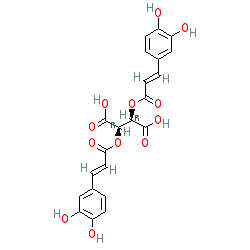SMILES: C1=CC(=C(C=C1/C=C/C(=O)O[C@@H](C(=O)O)[C@@H](OC(=O)/C=C/C2=CC(=C(C=C2)O)O)C(=O)O)O)O
InChIKey: YDDGKXBLOXEEMN-IABMMNSOSA-N

–EOF–